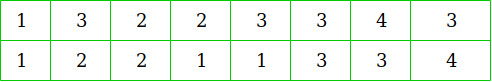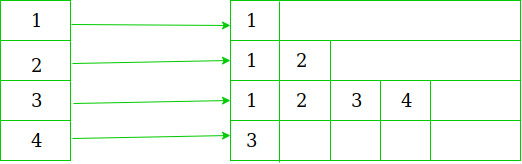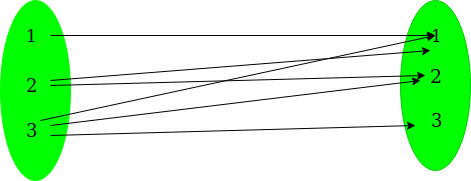# Convert many to many mappings to maximum no of one to one mappings

Given an array arr1 containing integers from 1..N and another array arr2 containing integers from 1..M. Each element of arr1 is mapped to more than one element in arr2. The task is to maximize the number of one to one mappings possible between both arrays.

Here one to one mappings means a unique element of arr1 must be mapped to unique element of arr2.
Mapping is given in the form of vector of pairs V where V.first() denotes elements of arr1 and V.second() denotes elements of arr2.

Examples:

```Input:  N = 3, M = 3
arr1[] = {1, 2, 3}
arr2[] = {1, 2, 3}
V = { {1, 1}, {2, 1}, {2, 2},
{3, 1}, {3, 2}, {3, 3} }
Output: 3
Explanation:
If we carefully see here mappings are
1 -------->  {1}
2 -------->  {1, 2}
3 -------->  {1, 2, 3}
so, maximum number of unique pairings
can be done as
1 -------->  {1}
2 -------->  {2}
3 -------->  {3}

Input: N = 3, M = 1
V = { {1, 1}, {2, 1}, {3, 1} };
Output: 1
Explanation:
Either 1 can be mapped or 2 or 3.
so maximum one mapping is possible.
```

Approach: The approach used here is Greedy.
Preprocessing step
We are given a vector of pairs which contains mapping information in an unsorted way for eg-so, we convert this mapping information into a vector of sets mapping.
For eg:This is representing the information in one to many mapping form.
This can be easily understood by the below diagramSteps:

1. Find the element in arr1[] which has least number of mappings with elements of arr2[].
2. Map this element to first element of its corresponding set.
3. Delete all the mappings of this element.
4. Also delete all the mappings which contain element from arr2[] those are already mapped.
5. Repeat these four steps for all the elements of arr1[].

Below is the implementation of Above approach:

 `// C++ Program to convert many to many mapping ` `// to maximum no of one to one mappings ` ` `  `#include ` `using` `namespace` `std; ` ` `  `// Returns the maximum number ` `// of one to one mappings between two arrays ` `int` `FindMax(vector >& V, ``int` `N, ``int` `M) ` `{ ` `    ``int` `ans = 0; ` ` `  `    ``// Stores whether an element ` `    ``// from the first array is used in mapping ` `    ``bool``* is_paired = ``new` `bool``[N]; ` ` `  `    ``// Contains mapping in sorted form ` `    ``vector > mapping(N + 1); ` ` `  `    ``// Initialize all the elements ` `    ``// of first array unused ` `    ``memset``(is_paired, ``sizeof``(is_paired), ``false``); ` ` `  `    ``// Insert mappings in sorted form ` `    ``for` `(``int` `i = 0; i < V.size(); i++) { ` `        ``mapping[V[i].first].insert(V[i].second); ` `    ``} ` ` `  `    ``// While there is always at least one element ` `    ``// which can be mapped to a unique element ` `    ``while` `(``true``) { ` `        ``int` `lowest = -1; ` ` `  `        ``// Finds element to be mapped ` `        ``for` `(``int` `i = 1; i <= N; i++) { ` ` `  `            ``// There is mapping possible ` `            ``if` `(mapping[i].size() > 0) { ` `                ``if` `(lowest == -1) ` `                    ``lowest = i; ` `                ``else` `if` `(mapping[i].size() ` `                         ``< mapping[lowest].size()) ` `                    ``lowest = i; ` `            ``} ` `        ``} ` ` `  `        ``if` `(lowest == -1) ` `            ``break``; ` ` `  `        ``// Element used in the mapping ` `        ``is_paired[lowest] = ``true``; ` ` `  `        ``int` `remove` `= *mapping[lowest].begin(); ` ` `  `        ``// Delete all the mappings of used element ` `        ``for` `(``int` `i = 1; i <= N; i++) { ` ` `  `            ``mapping[i].erase(``remove``); ` `            ``if` `(i == lowest) { ` `                ``mapping[i].clear(); ` `            ``} ` `        ``} ` `        ``ans++; ` `    ``} ` `    ``return` `ans; ` `} ` ` `  `// Main Function ` `int` `main() ` `{ ` `    ``int` `N = 3; ` `    ``int` `M = 3; ` `    ``int` `arr1[] = { 1, 2, 3 }; ` `    ``int` `arr2[] = { 1, 2, 3 }; ` ` `  `    ``vector > ` `        ``V{ { 1, 1 }, { 2, 1 }, { 2, 2 }, { 3, 1 }, { 3, 2 }, { 3, 3 } }; ` ` `  `    ``cout << FindMax(V, N, M) << endl; ` ` `  `    ``return` `0; ` `} `

Output:

```3
```

Time Complexity:where x is maximum no one to one mappings.

My Personal Notes arrow_drop_upCheck out this Author's contributed articles.

If you like GeeksforGeeks and would like to contribute, you can also write an article using contribute.geeksforgeeks.org or mail your article to contribute@geeksforgeeks.org. See your article appearing on the GeeksforGeeks main page and help other Geeks.

Please Improve this article if you find anything incorrect by clicking on the "Improve Article" button below.

Article Tags :
Practice Tags :

Be the First to upvote.

Please write to us at contribute@geeksforgeeks.org to report any issue with the above content.